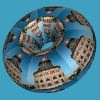Union College Department of Mathematics WeBWorK Homework Server

WeBWorK courses for Winter term 2023:

 Math 110 - 1 & 2 Calculus I Prof. Plofker Math 112 - 1 & 2 Calculus II Prof. Qian Math 112 - 3 Calculus II Prof. Malen Math 112 - 4 Calculus II Prof. Gajek-Leonard Math 112 - 5 Calculus II Prof. Hatley Math 113 - 1 Accelerated Single-Variable Calculus Prof. Lesh Math 115 - 1 & 2 Calculus III Prof. Friedman Math 115 - 3 Calculus III Prof. Johnson Math 117 - 1 Calculus IV Prof. Gasparovic Math 234 - 1 Differential Equations Prof. Wang IMP 120 - 1 Integrated Math and Physics I Prof. Tonnesen-Friedman

Courses from past terms
An Introduction to WeBWork for Students

Union College Department of Mathematics